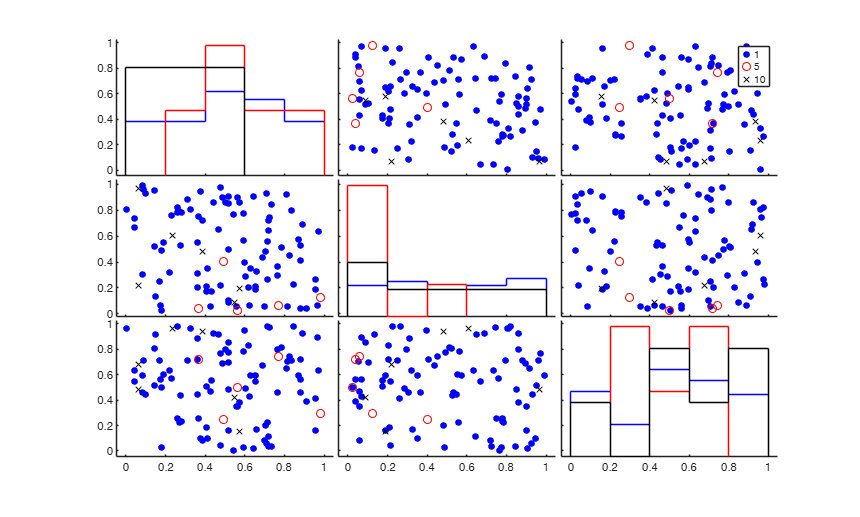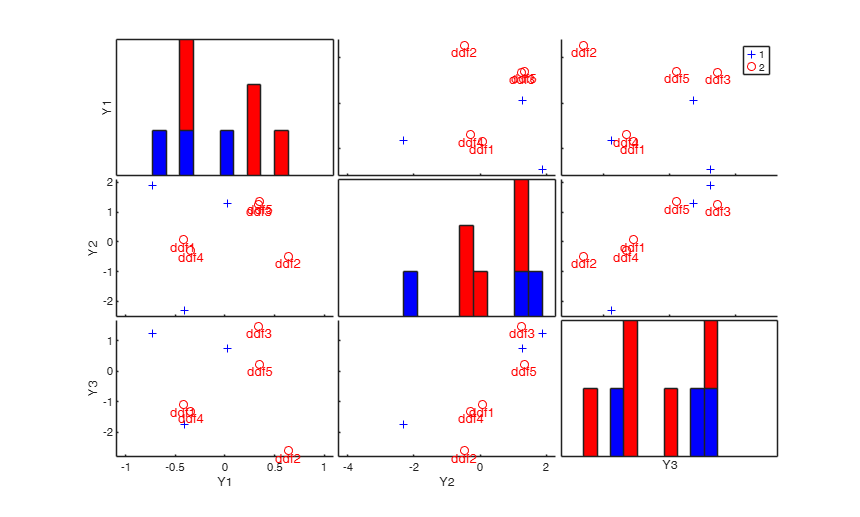# Documentation

add2spm adds objects (personalized clickable multilegends and text labels) to the scatter plot matrix

## Syntax

• add2spm(H,AX,BigAx)example
• add2spm(H,AX,BigAx,Name,Value)example

## Description

 add2spm(H, AX, BigAx) add2spm with all default options.

 add2spm(H, AX, BigAx, Name, Value) add2spm with optional arguments.

## Examples

expand all

###add2spm with all default options.

load fisheriris;
% Create scatter plot matrix with specific legends
[H,AX,BigAx]=gplotmatrix(meas,[],species);
% The legens are not clickable
% [H,AX,BigAx]=gplotmatrix(meas,[],species,[],[],[],[],'grpbars')
% Now the legends become clickableUse of userleg variable. Set some multivariate data and some groups.

y = randn(100,3);
group = ones(100,1); group(1:10,1) = 444; group(11:20,1) = 777;
% Make a scatterplot using gplotmatrix defaults.
% The legends automatically created are '1','444' and '777'.
[H,AX,BigAx] = gplotmatrix(y,[],group,'brk','.ox');
% with add2spm with default options, the gplotmatrix legends
% will become clickable.
% by running add2spm with option 'userleg' set to '1', and by setting
% the figure Tag to a string containing the word 'group', the clickable
% legends will become 'group 1', 'group 2' and 'group 3'.
[H,AX,BigAx] = gplotmatrix(y,[],group,'brk','.ox');
set(gcf, 'Tag', 'this is a string with group')
% by running add2spm with 'userleg', {'my group 1' ; 'my group 4' ; 'my
% group 7'} the legends change as desired.
[H,AX,BigAx] = gplotmatrix(y,[],group,'brk','.ox');
% Now create a group on units contaminated by outliers. By running
% add2spm with option 'userleg' set to '1', and by setting the figure
% Tag to a string containing the word 'outlier', the clickable legends
% become 'normal units' and 'outliers'. Remark: the keep the proper
% order of the legends, it is sufficient to reserve for the outlier id
% variable the largest id number.
y = randn(100,2); y(25:35,:) = y(25:35,:) + 10;
group = ones(100,1);group(25:35,1) = 5;
figure('Tag','this is a dataset with outliers');
[H,AX,BigAx] = gplotmatrix(y,[],group,'br','ox');
add2spm(H,AX,BigAx,'userleg','1');

## Related Examples

expand all

###Example of use of option 'labeladd'.

close all;
% 'labeladd' is set to '1' to add labels found in the UserData
% property of the last group in each panel of the scatter matrix.
% It can be retrieved from H(1,end,end) (i.e. first row, last column,
% last group of the scatter matrix handles).
y = rand(100,3);
group = ones(100,1); group(1:5,1) = 5; group(10:15,1) = 10;
[H,AX,BigAx] = gplotmatrix(y,[],group,'brk','.ox');
% column vector of labels is set to the integers from 1 to size of last
% data group
labels = (1:numel(get(H(1,end,end),'YData')))';
% assign labels to the 'UserData' property of the last data group
set(H(:,:,1), 'UserData' , labels);
add2spm(H,AX,BigAx,'labeladd','1');###Example of use of option 'labeladd' combined with 'RowNamesLabels'.

close all;
n=8;
Y = randn(n,3);
group = ones(n,1);
group(1:5) = 2;
[H,AX,BigAx] = spmplot(Y,group);
% Create cell containing the name of the rows.
label={'ddf1' 'ddf2' 'ddf3' 'ddf4' 'ddf5' 'ddf6' 'ddf7' 'ddf8'};
% Labels are added to the units which belong to group 2 (that is to the
% first 5 units). The labels are taken from cell label
add2spm(H,AX,BigAx,'labeladd','1','RowNamesLabels',label);## Input Arguments

### H — handles to the lines on the graphs. 2D or 3D array.

H is an array of handles to the lines on the graphs. The array's third dimension corresponds to groups in the grouping variable. For more details see the output arguments of gplotmatrix.

Data Types: single| double

### AX — handles to the axes of the individual plots. Matrix.

AX is a matrix of handles to the axes of the individual plots.

For more details see the output arguments of gplotmatrix.

Data Types: single| double

### BigAx — handle to big (invisible) axes framing the entire plot matrix. Scalar.

For more details see the output arguments of gplotmatrix.

Data Types: single| double

### Name-Value Pair Arguments

Specify optional comma-separated pairs of Name,Value arguments. Name is the argument name and Value is the corresponding value. Name must appear inside single quotes (' '). You can specify several name and value pair arguments in any order as  Name1,Value1,...,NameN,ValueN.

Example:  'labeladd','1' , 'userleg','1' , 'RowNamesLabels',{'a' 'bb' 'cc' 'mmm'} 

### labeladd —add labels for a group of units.char.

Default is '', i.e. no labels are added to the symbols inside each scatter.

If set to '1', add labels to the units of the last data group (or the group with the largest value of the grouping variable) in each panel of the scatter matrix. The labels which are added are based on the content of the 'UserData' field of the last group. This can be achieved by means of instruction set(H(:,:,end), 'UserData' , unit_labels), where unit_labels is a column vector of numbers or strings.

See last example below for a concrete case.

Example:  'labeladd','1' 

Data Types: char

### userleg —user legend.char.

It is used to control the legend of the plot.

- Default is ''. In this case, existing legends are left as they are and simply made clickable; however, if there is no legend, a default one is created using the syntax 'Group 1', 'Group 2', etc.

- If it is set to '1', the legends are updated depending on the context of use and are made clickable. The context is determined by the occurence of specific words in the Tag of the current figure. The strings/contexts currently addressed are: 'outlier' (for 'Outliers' and 'Normal units'), 'brush' (for 'Brushed units 1', 'Brushed units 2', etc.), 'group' (for 'Group 1', 'Group 2', etc.), '' i.e. the Tag of the figure is not defined;

in this case the legend takes the values in the DisplayName property of the scatter matrix. This is determined by the 'group' option of the spmplot or gplotmatrix functions.

- If it is a cell of strings, e.g. {'FIAT' ; 'BMW' ; 'VOLVO'}, then such strings are used for the legend.

Example:  'userleg','1' 

Data Types: char

### RowNamesLabels —cell of length n, where n is the number of points in each scatter, containing the labels of the units.if this field is empty the sequence 1:n will be used to label the units.

Example:  'RowNamesLabels',{'a' 'bb' 'cc' 'mmm'} 

Data Types: cell

## Output Arguments

add2spm is essentially used within FSDA function spmplot. However its logic can be also demonstrated with MATLAB function gplotmatrix, as in the examples below.

As default add2spm makes legends in the existing scatter plot matrix clickable or creates a clickable multilegend if the legend does not exist.

Using varargin it is possible to: 1. Personalize the legend of groups in the scatterplot matrix. See option 'userleg'.

2. Add labels of the units belonging to the last data group (or to the group with the largest value in the grouping variable) of each scatter (panel). See option 'labeladd'.

Tufte E.R. (1983), "The visual display of quantitative information", Graphics Press, Cheshire.